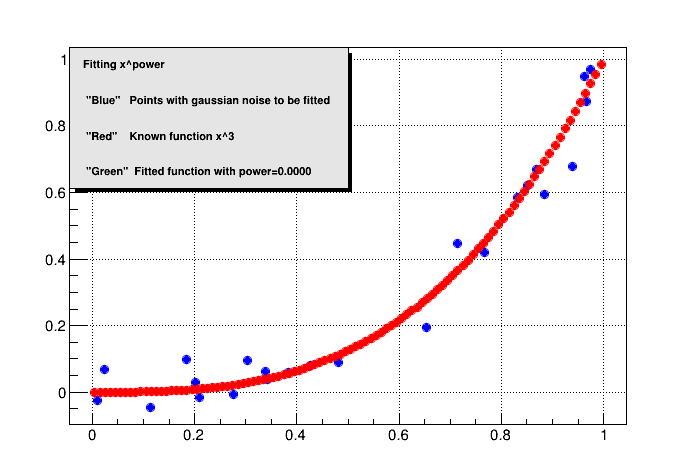# Simple Fitting¶

Create an exponential fitting The idea is to create a set of numbers x,y with the function x^3 and some noise from ROOT, fit the function to get the exponent (which must be near 3) and plot the points with noise, the known function and the fitted function

Author: Omar Zapata
This notebook tutorial was automatically generated with ROOTBOOK-izer from the macro found in the ROOT repository on Sunday, September 19, 2021 at 08:24 AM.

In :
%%cpp -d
#include<TRInterface.h>
#include<TRandom.h>

In file included from input_line_42:1:
In file included from /home/sftnight/build/workspace/root-makedoc-master/rootspi/rdoc/src/master.build/include/TRInterface.h:15:
In file included from /home/sftnight/build/workspace/root-makedoc-master/rootspi/rdoc/src/master.build/include/TRObject.h:14:
#include<RcppCommon.h>
^~~~~~~~~~~~~~

In :
TCanvas *c1 = new TCanvas("c1","Curve Fitting",700,500);
c1->SetGrid();


Draw a frame to define the range

In :
TMultiGraph *mg = new TMultiGraph();


Create the first plot (points with gaussian noise)

In :
const Int_t n = 24;
Double_t x1[n] ;
Double_t y1[n] ;


Generate the points along a x^3 with noise

In :
TRandom rg;
rg.SetSeed(520);
for (Int_t i = 0; i < n; i++) {
x1[i] = rg.Uniform(0, 1);
y1[i] = TMath::Power(x1[i], 3) + rg.Gaus() * 0.06;
}

TGraph *gr1 = new TGraph(n,x1,y1);
gr1->SetMarkerColor(kBlue);
gr1->SetMarkerStyle(8);
gr1->SetMarkerSize(1);

// create the second plot
TF1 *f_known=new TF1("f_known","pow(x,3)",0,1);
TGraph *gr2 = new TGraph(f_known);
gr2->SetMarkerColor(kRed);
gr2->SetMarkerStyle(8);
gr2->SetMarkerSize(1);


Passing data to rfot fitting

In :
ROOT::R::TRInterface &r=ROOT::R::TRInterface::Instance();
r["x"]<<TVectorD(n, x1);
r["y"]<<TVectorD(n, y1);

In file included from libRInterface dictionary payload:5:
#include<RcppCommon.h>
^~~~~~~~~~~~~~
Error in <TInterpreter::AutoParse>: Error parsing payload code for class ROOT::R::TRInterface with content:

#define _BACKWARD_BACKWARD_WARNING_H
#include "RExports.h"
#include "TRDataFrame.h"
#include "TRFunctionExport.h"
#include "TRFunctionImport.h"
#include "TRInterface.h"
#include "TRInternalFunction.h"
#include "TRObject.h"

#undef  _BACKWARD_BACKWARD_WARNING_H

input_line_56:2:35: error: incomplete type 'ROOT::R::TRInterface' named in nested name specifier
ROOT::R::TRInterface &r=ROOT::R::TRInterface::Instance();
~~~~~~~~~^~~~~~~~~~~~~
libRInterface dictionary forward declarations' payload:9:93: note: forward declaration of 'ROOT::R::TRInterface'
namespace ROOT{namespace R{class __attribute__((annotate("$clingAutoload$TRInterface.h")))  TRInterface;}}
^
input_line_56:3:2: error: no viable overloaded operator[] for type 'ROOT::R::TRInterface'
r["x"]<<TVectorD(n, x1);
~^~~~
input_line_56:4:2: error: no viable overloaded operator[] for type 'ROOT::R::TRInterface'
r["y"]<<TVectorD(n, y1);
~^~~~


Creating a r data frame

In :
r<<"ds<-data.frame(x=x,y=y)";

input_line_60:2:3: error: use of undeclared identifier 'r'
(r << "ds<-data.frame(x=x,y=y)")
^
Error in <HandleInterpreterException>: Error evaluating expression (r << "ds<-data.frame(x=x,y=y)").
Execution of your code was aborted.


Fitting x and y to x^power using nonlinear least squares

In :
r<<"m <- nls(y ~ I(x^power),data = ds, start = list(power = 1),trace = T)";

input_line_62:2:3: error: use of undeclared identifier 'r'
(r << "m <- nls(y ~ I(x^power),data = ds, start = list(power = 1),trace = T)")
^
Error in <HandleInterpreterException>: Error evaluating expression (r << "m <- nls(y ~ I(x^power),data = ds, start = list(power = 1),trace = T)").
Execution of your code was aborted.


Getting the exponent

In :
Double_t power;
r["summary(m)$coefficients"]>>power; TF1 *f_fitted=new TF1("f_fitted","pow(x,)",0,1); f_fitted->SetParameter(0,power);  input_line_64:2:3: error: use of undeclared identifier 'r' (r["summary(m)$coefficients"] >> ((*(Double_t*)0x7f404d8cc258)))
^
Error in <HandleInterpreterException>: Error evaluating expression (r["summary(m)\$coefficients"] >> ((*(Double_t*)0x7f404d8cc258))).
Execution of your code was aborted.


Plotting the fitted function

In :
TGraph *gr3 = new TGraph(f_fitted);
gr3->SetMarkerColor(kGreen);
gr3->SetMarkerStyle(8);
gr3->SetMarkerSize(1);

mg->Draw("ap");

Error in <TGraph::TGraph>: Pointer to function is null


Displaying basic results

In :
TPaveText *pt = new TPaveText(0.1,0.6,0.5,0.9,"brNDC");
pt->SetFillColor(18);
pt->SetTextAlign(12);
pt->AddText(" \"Blue\"   Points with gaussian noise to be fitted");
TString fmsg;
fmsg.Form(" \"Green\"  Fitted function with power=%.4lf",power);
pt->Draw();
c1->Update();
return c1;

Error in <TGraphPainter::PaintGraph>: illegal number of points (0)


Draw all canvases

In :
gROOT->GetListOfCanvases()->Draw()Error in <TGraphPainter::PaintGraph>: illegal number of points (0)